×#### Thank you for registering.

One of our academic counsellors will contact you within 1 working day.

Click to Chat

1800-1023-196

+91-120-4616500

CART 0

• 0

MY CART (5)

Use Coupon: CART20 and get 20% off on all online Study Material

ITEM
DETAILS
MRP
DISCOUNT
FINAL PRICE
Total Price: Rs.

There are no items in this cart.
Continue Shopping• Complete JEE Main/Advanced Course and Test Series
• OFFERED PRICE: Rs. 15,900
• View Details

```Chapter 13: Complex Numbers – Exercise 13.2

Complex Numbers – Exercise 13.2 – Q.1(i)

(1 + i)(1 + 2i) = 1 × (1 +2i) + i(1 + 2i)

= 1 + 2i + i + 2i2

= 1 + 3i - 2

= -1 + 3i

∴ (1 + i)(1 + 2i) = -1 + 3i

Complex Numbers – Exercise 13.2 – Q.1(ii)Complex Numbers – Exercise 13.2 – Q.1(iii)Complex Numbers – Exercise 13.2 – Q.1(iv)Complex Numbers – Exercise 13.2 – Q.1(v)Complex Numbers – Exercise 13.2 – Q.1(vi)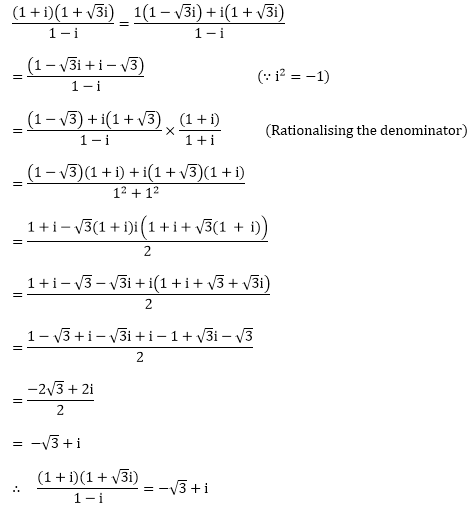Complex Numbers – Exercise 13.2 – Q.1(vii)Complex Numbers – Exercise 13.2 – Q.1(viii)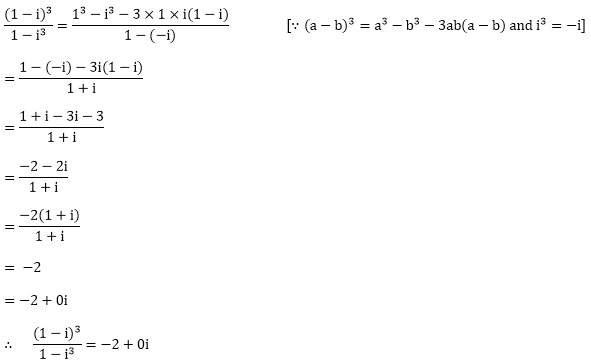Complex Numbers – Exercise 13.2 – Q.1(ix)Complex Numbers – Exercise 13.2 – Q.1(x)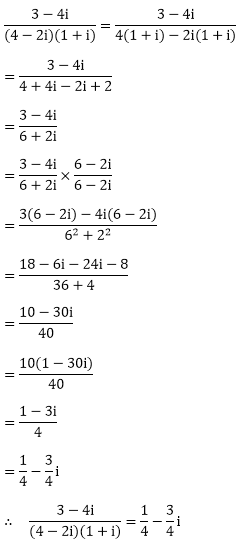Complex Numbers – Exercise 13.2 – Q.1(xi)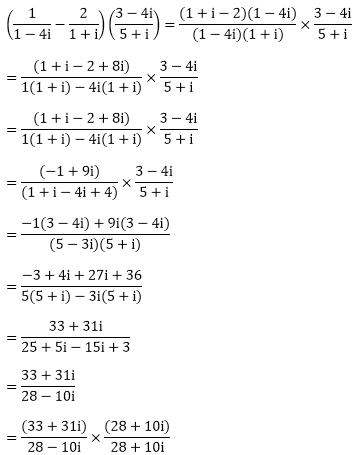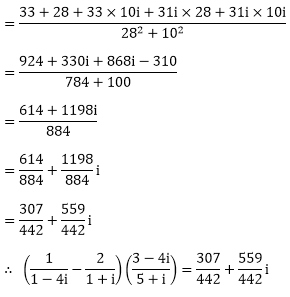Complex Numbers – Exercise 13.2 – Q.1(xii)

We have,Complex Numbers – Exercise 13.2 – Q.2(i)

We have (x + iy)(2 - 3i) = 4 + i

⟹ x(2 - 3i) + iy ((2 - 3i) = 4 + i

⟹ 2x - 3xi + 2yi + 3y = 4 + i

⟹ 2x + 3y + i (-3x + 2y) = 4 + i

Equating the real and imaginary parts we get

2x + 3y = 4        ......(i)

- 3x + 2y = 1         ......(ii)

Multiplying (i) by 3 and (ii) by 2 and adding

6x - 6x - 9y + 4y = 12 + 2

⟹ 13y = 14Substituting the value of y in (i), we getHenceComplex Numbers – Exercise 13.2 – Q.2(ii)

(3x - 2iy) (2 + i)2 = 10(1 + i)

⟹ (3x – 2iy)(22 + i2 + 2 × 2 × i) = 10 + 10i

⟹ (3x – 2iy)(4 – 1 + 4i) = 10 + 10i

⟹ 3x(3 + 4i) – 2iy(3 + 4i) = 10 + 10i

⟹ 9x + 12xi – 6yi + 8y = 10 + 10i

⟹ 9x + 8y+i(12x – 6y) = 10 + 10i
Equating the real andim aginary parts we get

9x + 8y = 10         ...........(i)

12x - 6y = 10       ...........(ii)

Multiplying (i) by 6 and (ii) by 8 and adding

54x + 96x + 48y – 48y =  60 + 80

⟹ 150x = 140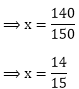Substituting value of x in (i) we get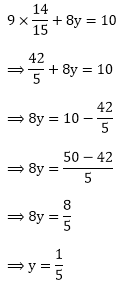```### Course Features

• 728 Video Lectures
• Revision Notes
• Previous Year Papers
• Mind Map
• Study Planner
• NCERT Solutions
• Discussion Forum
• Test paper with Video Solution